Learn all Concepts of Chapter 8 Class 10 (with VIDEOS). Check - Trigonometry - Class 10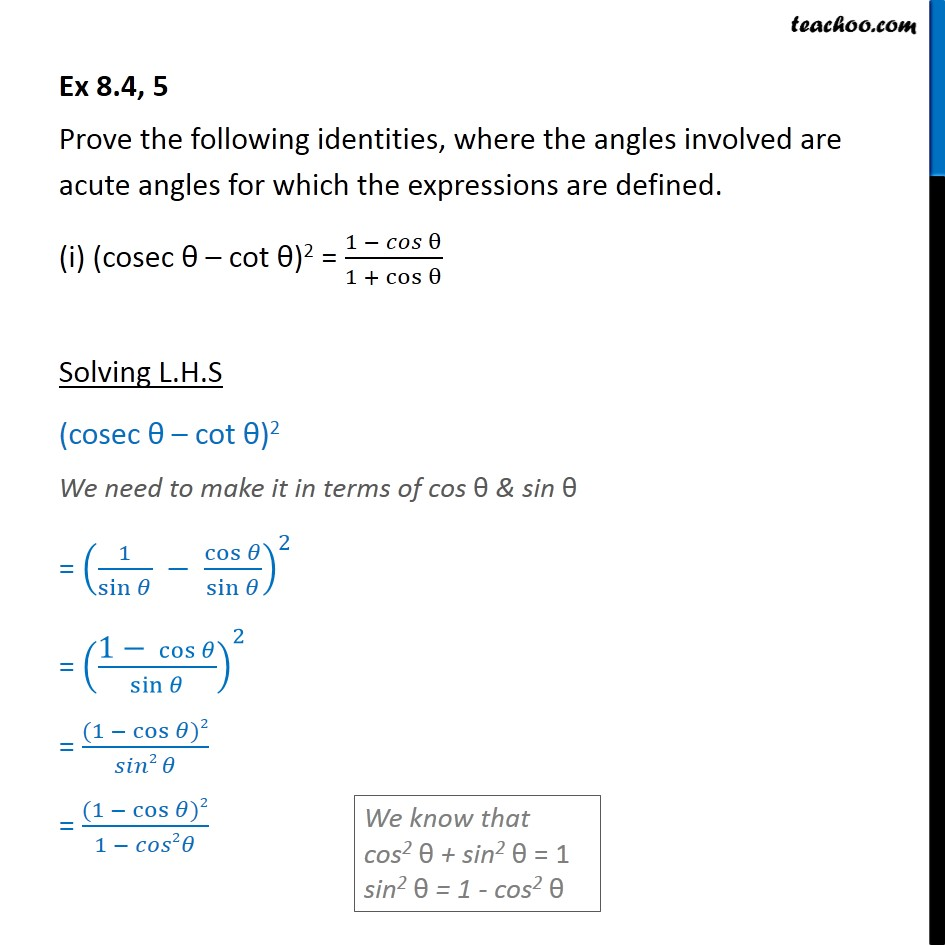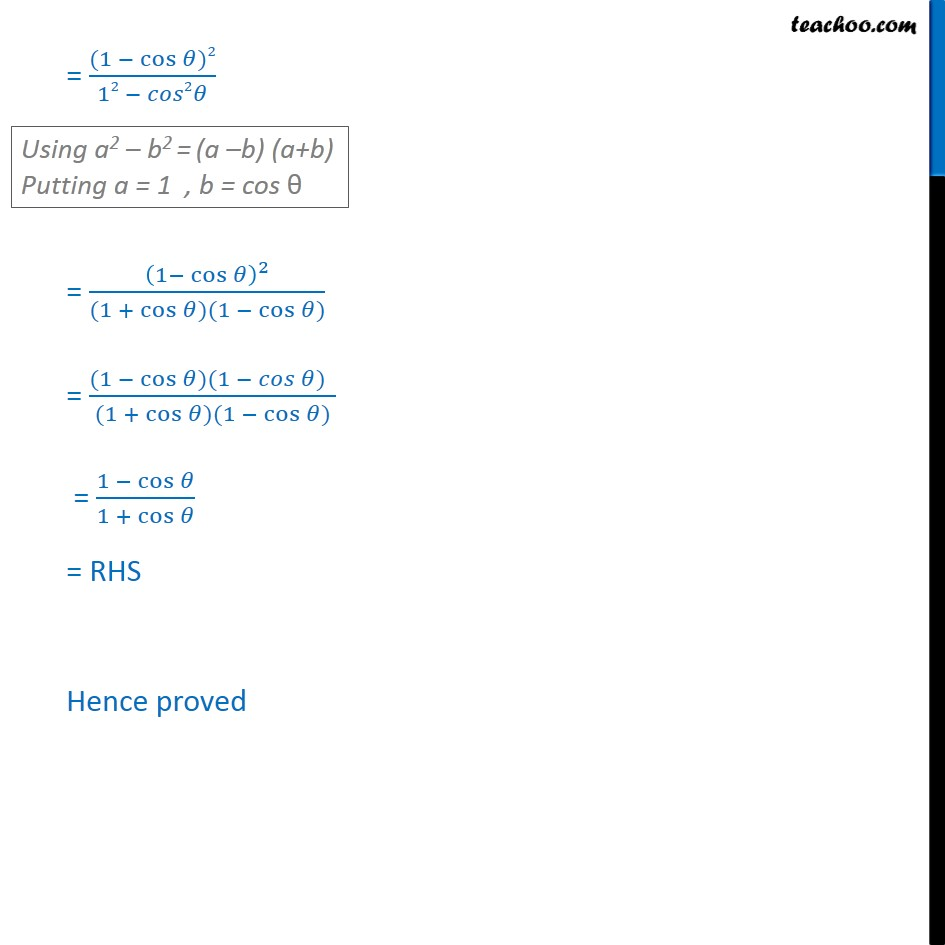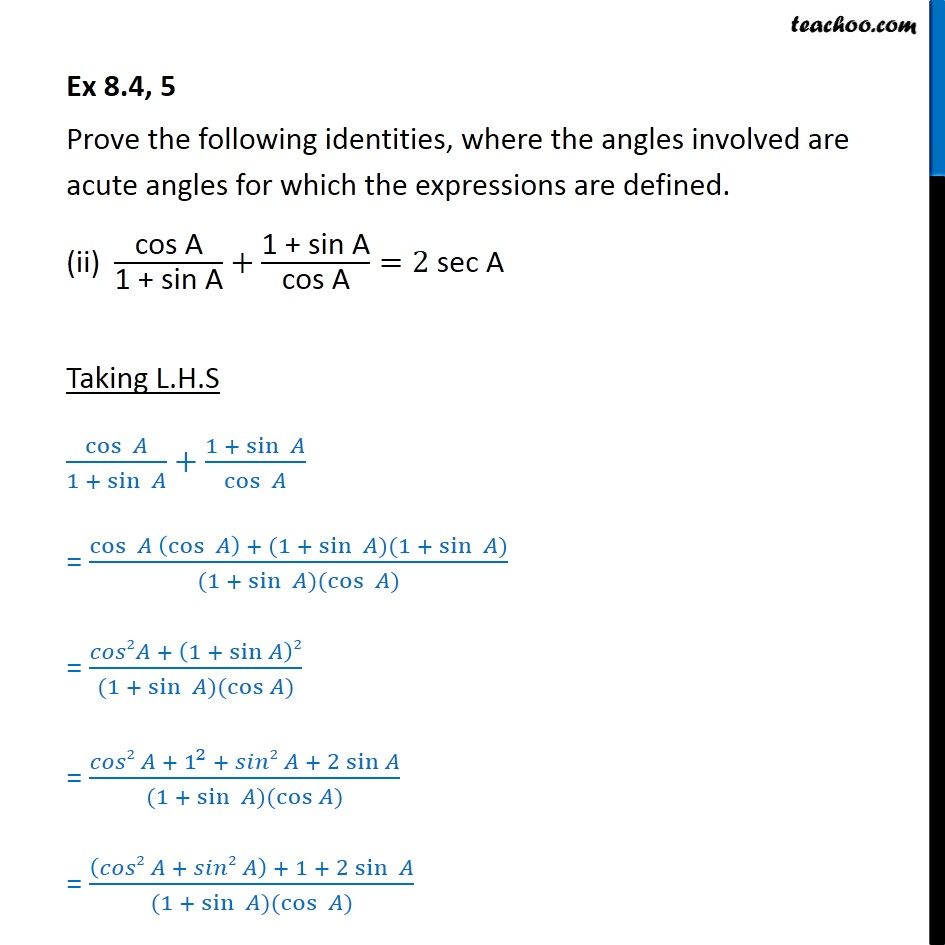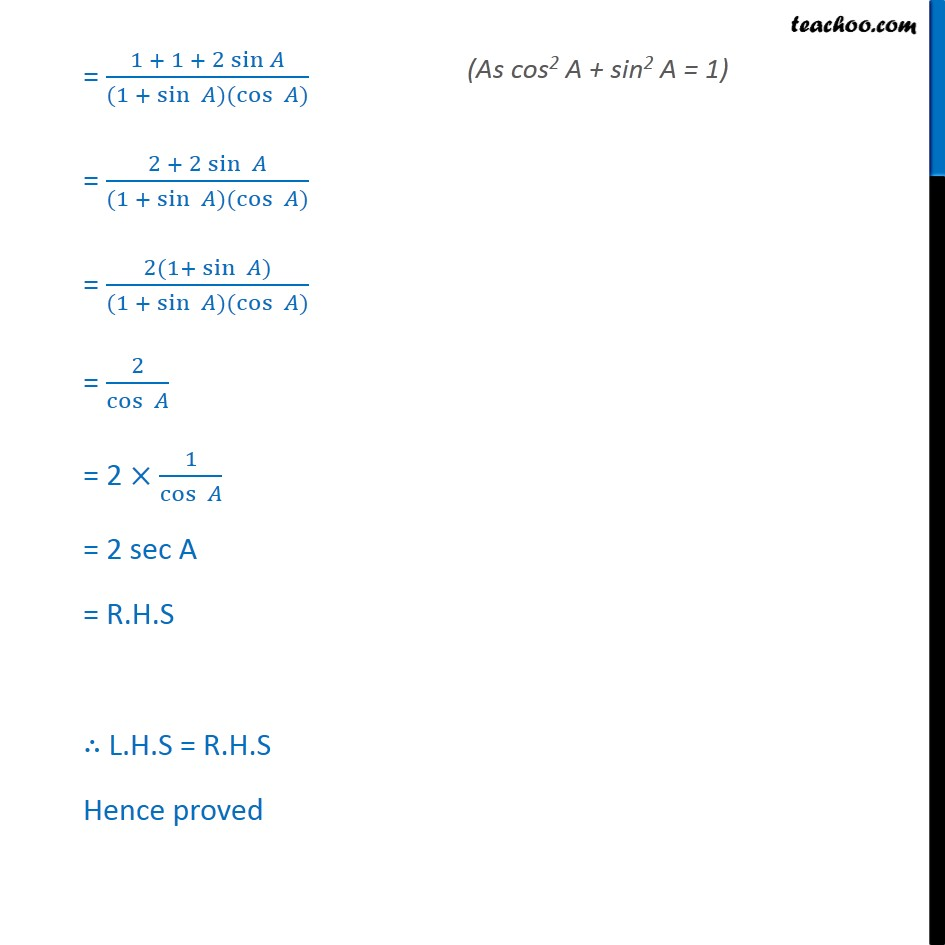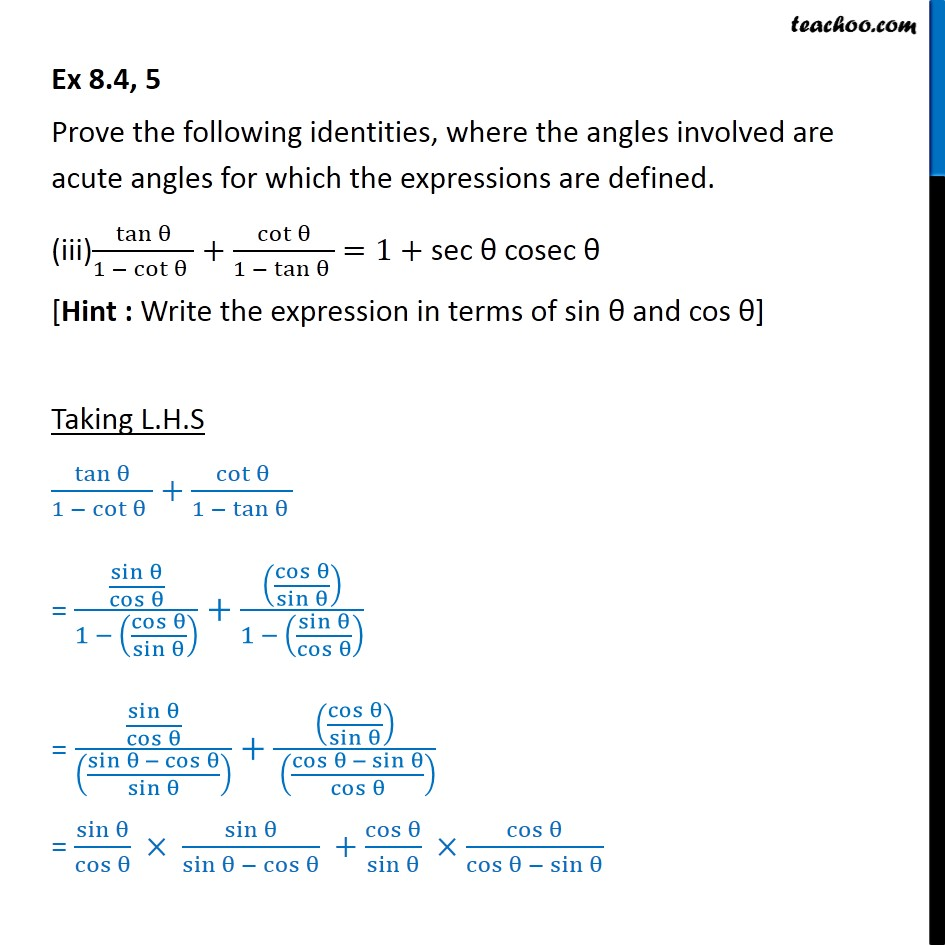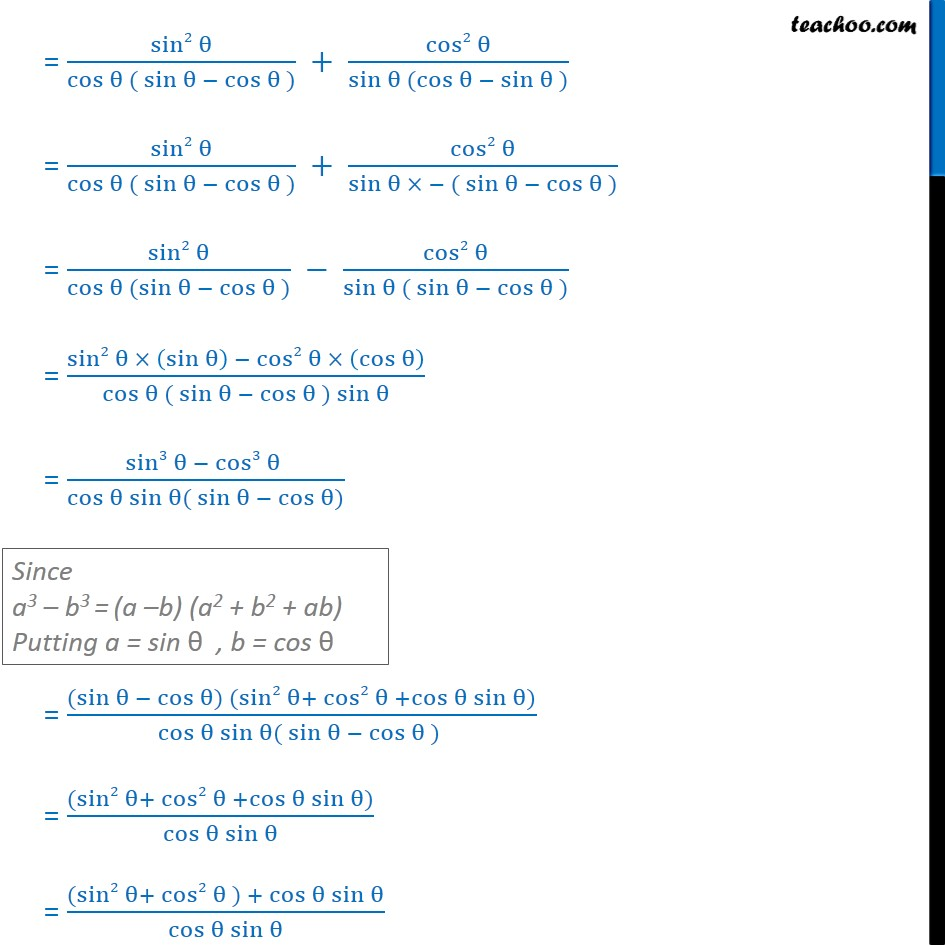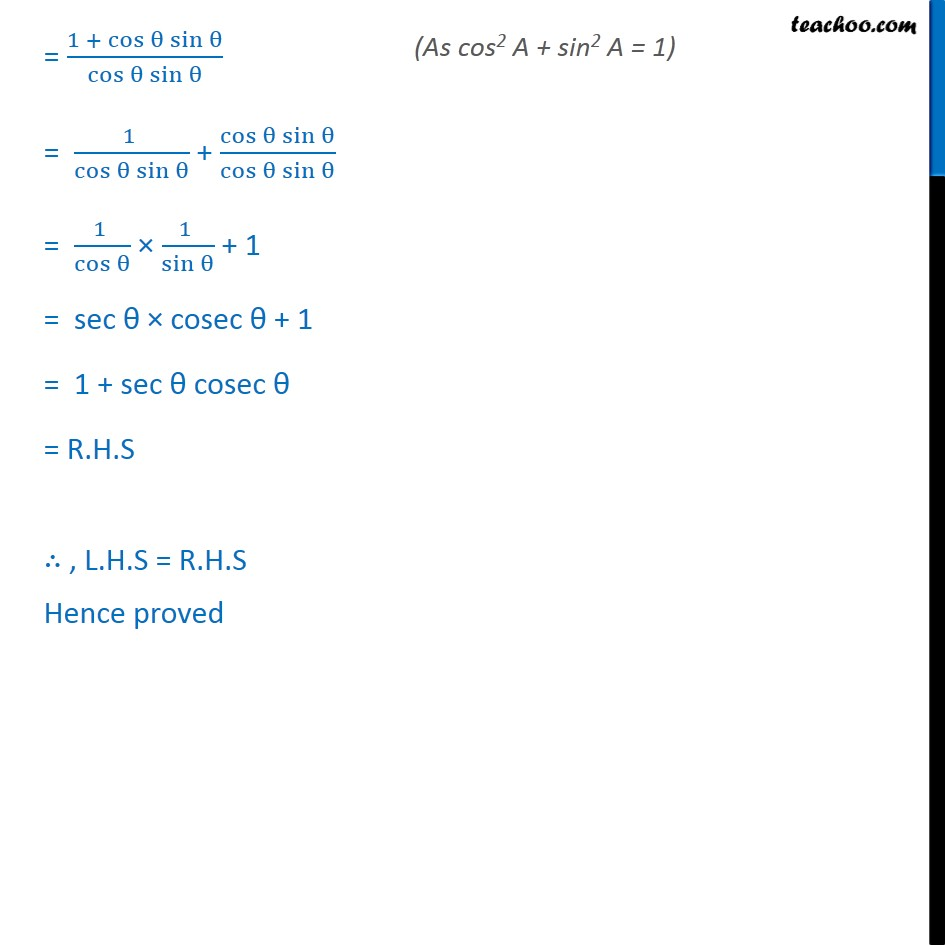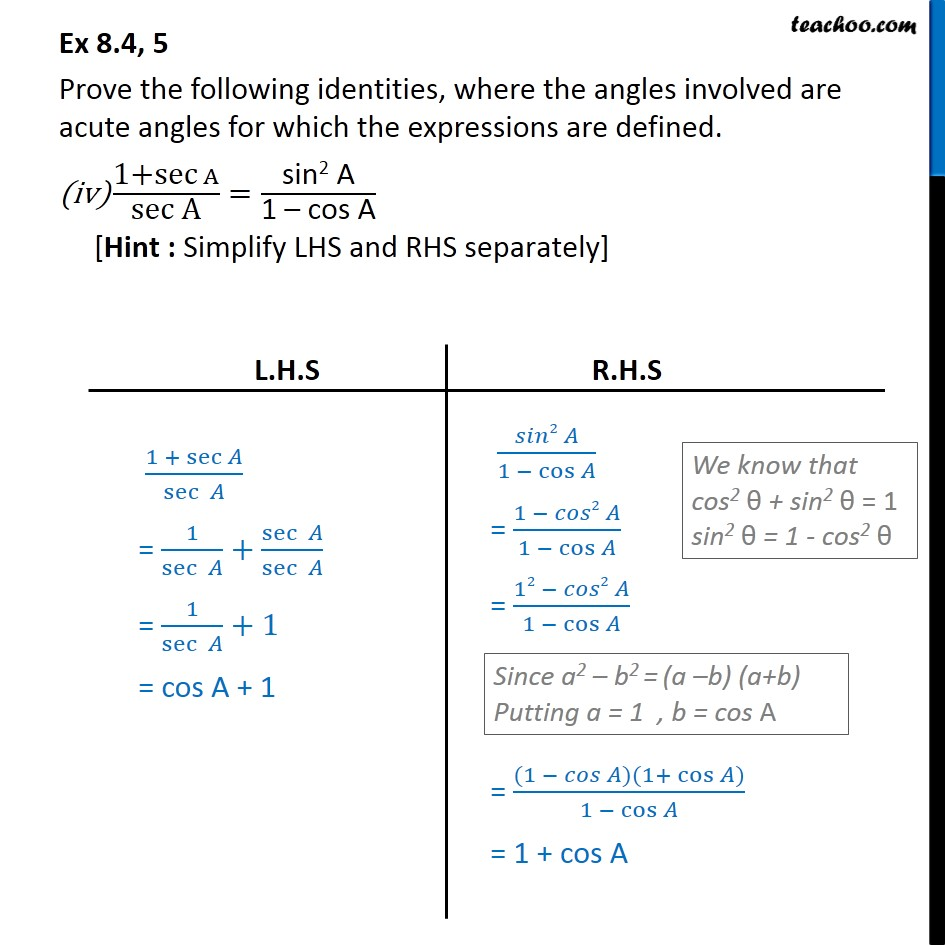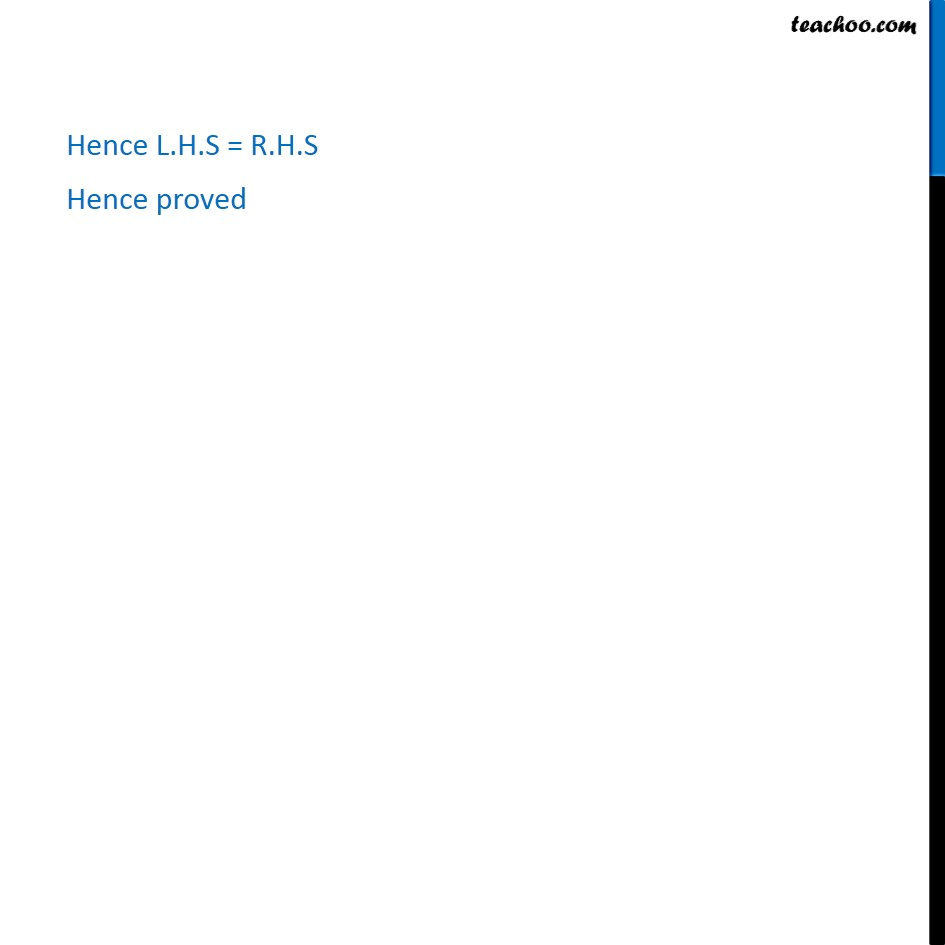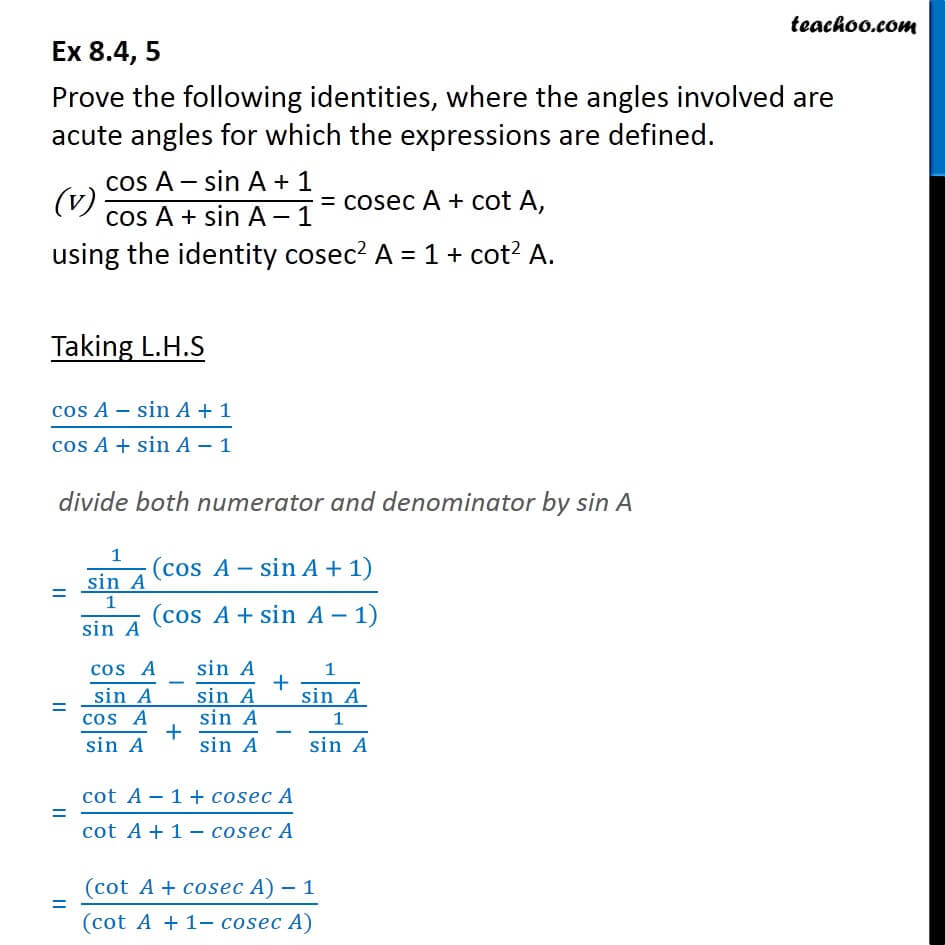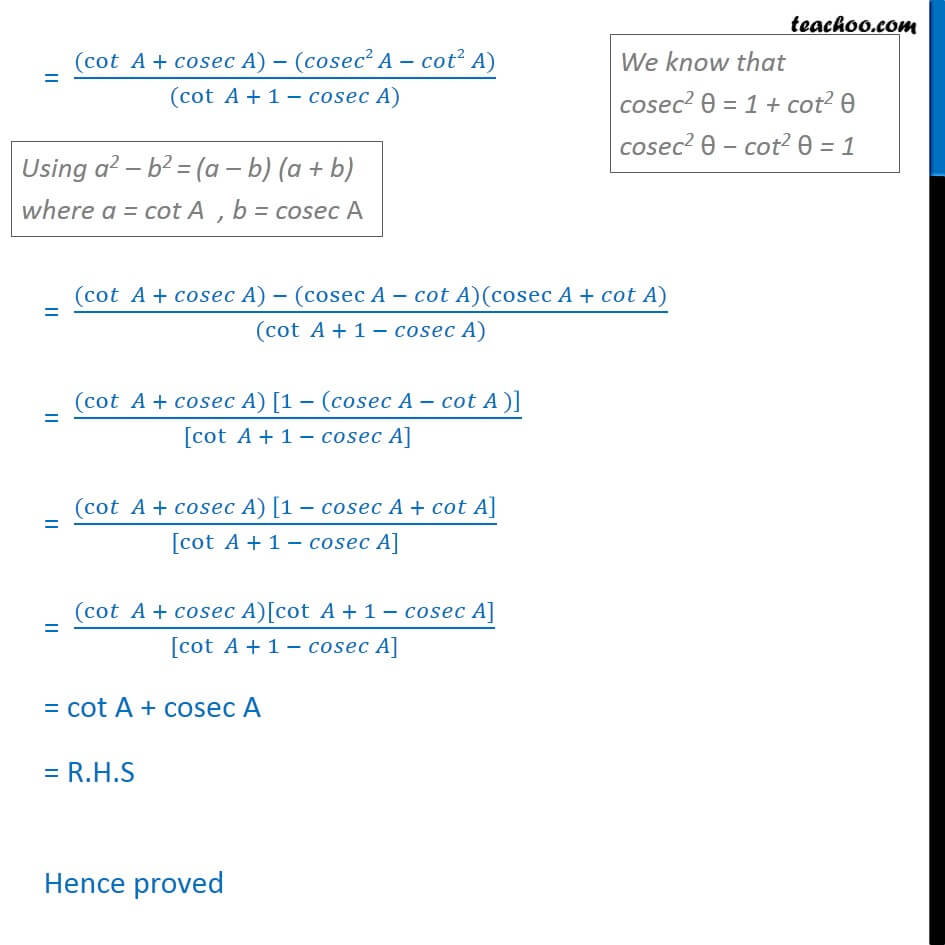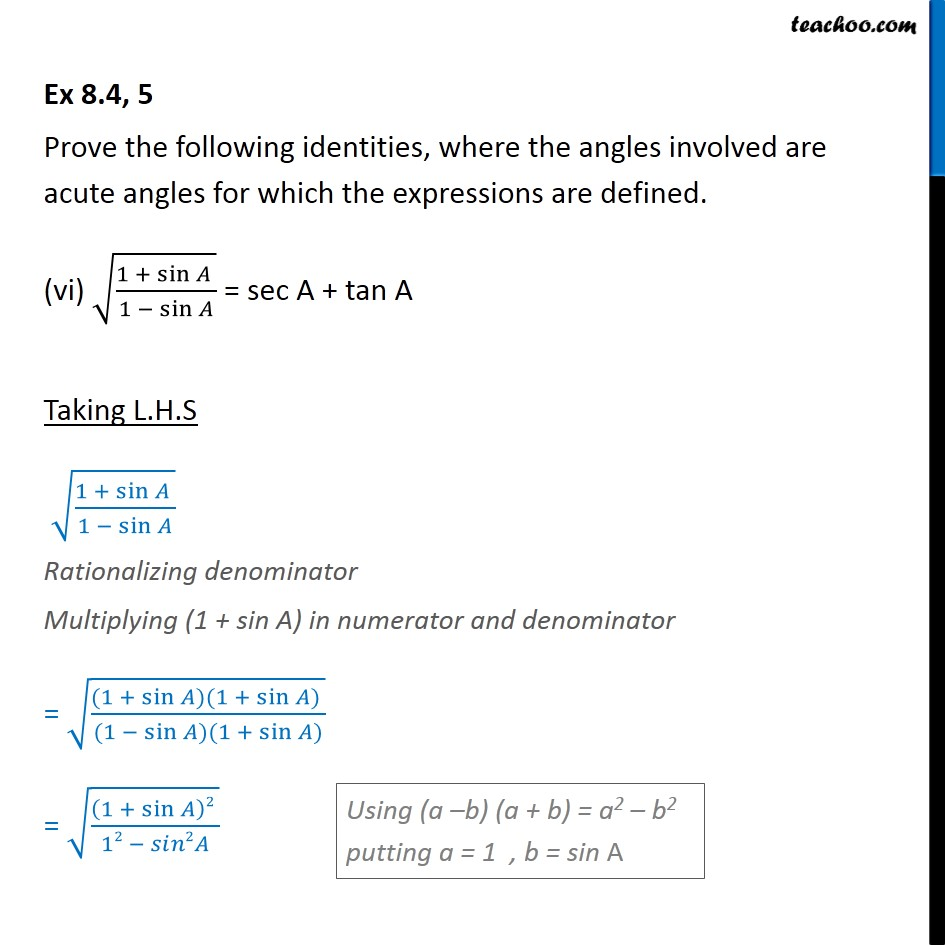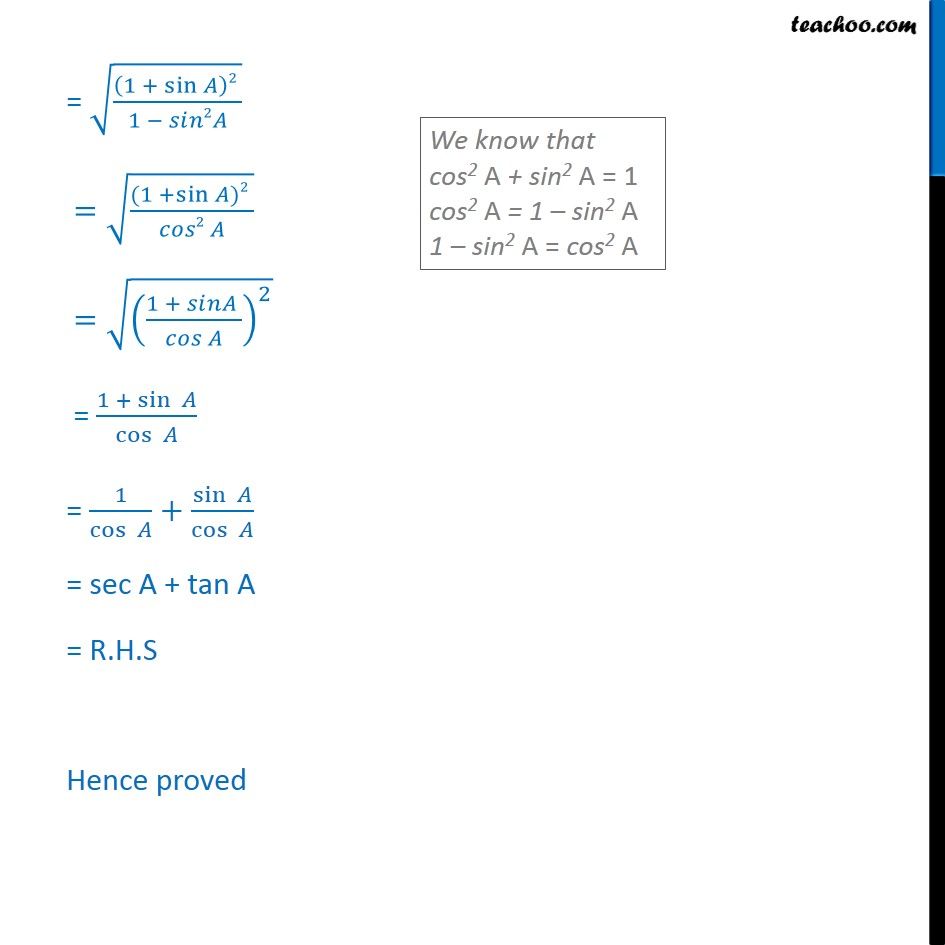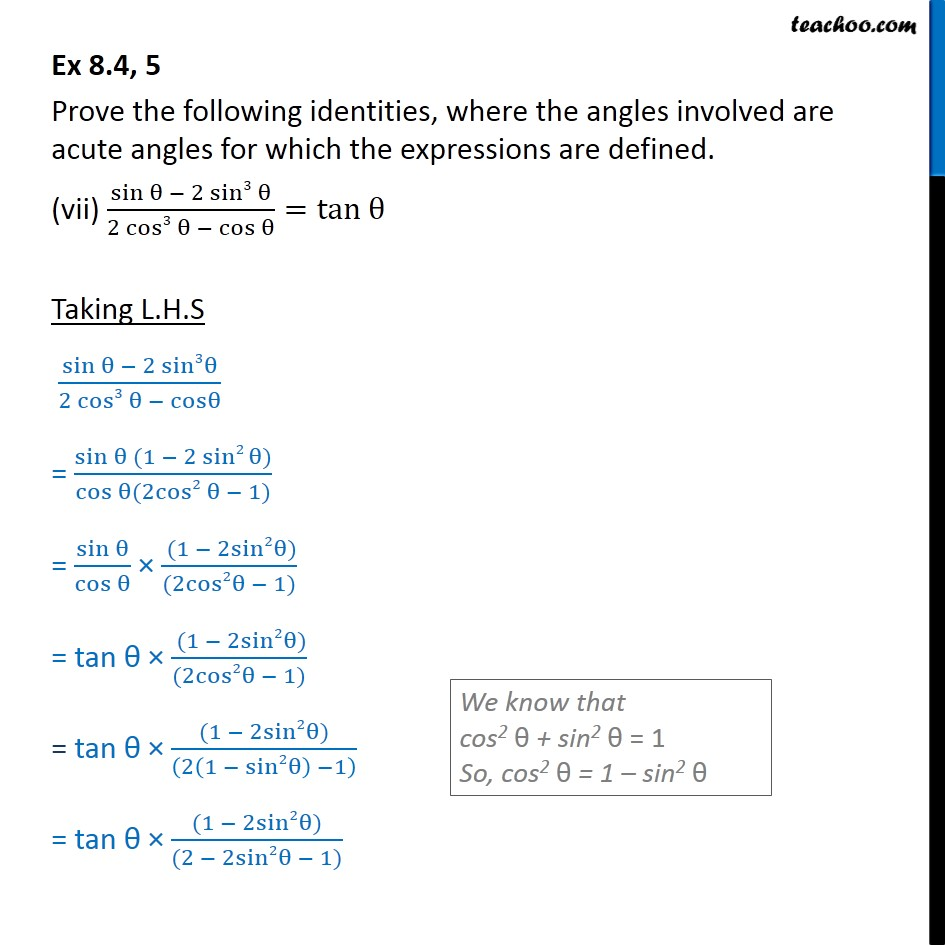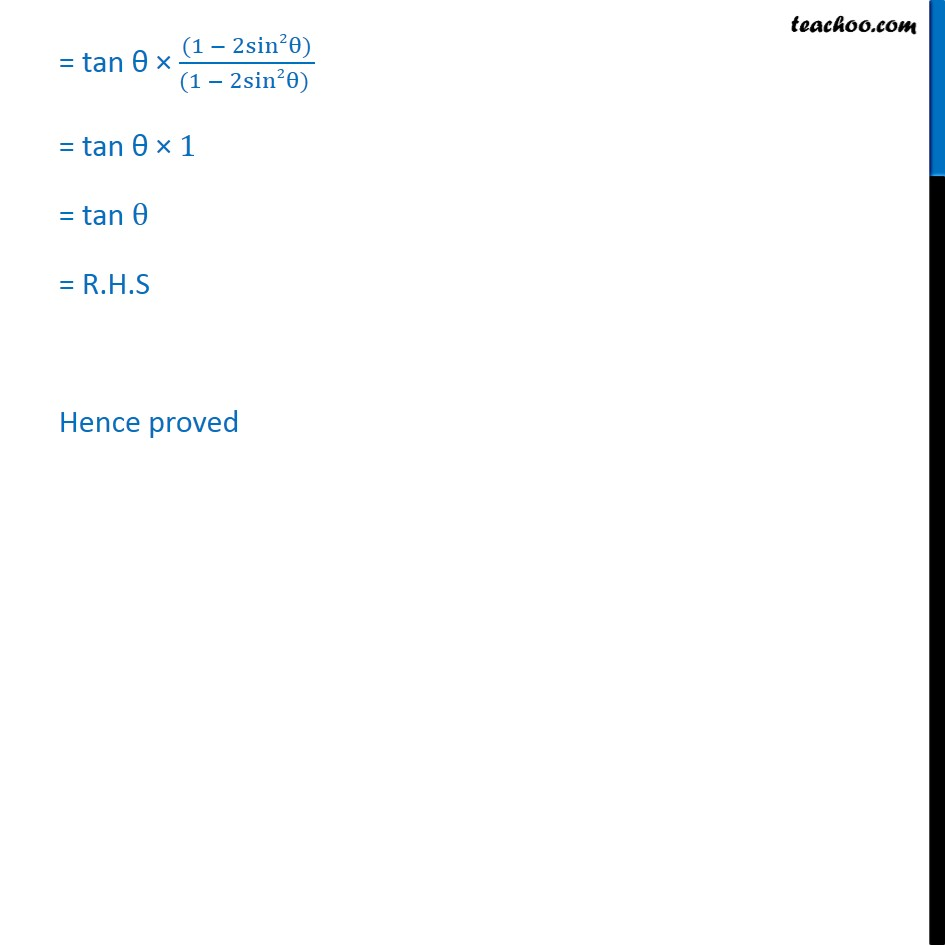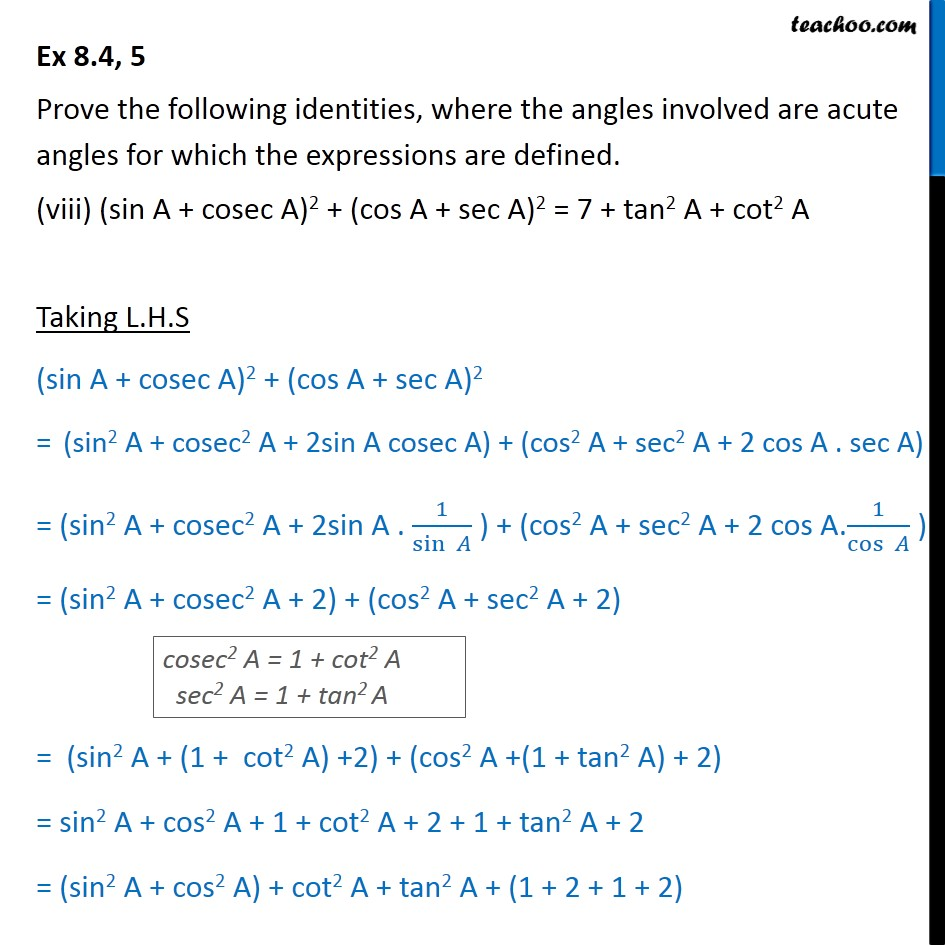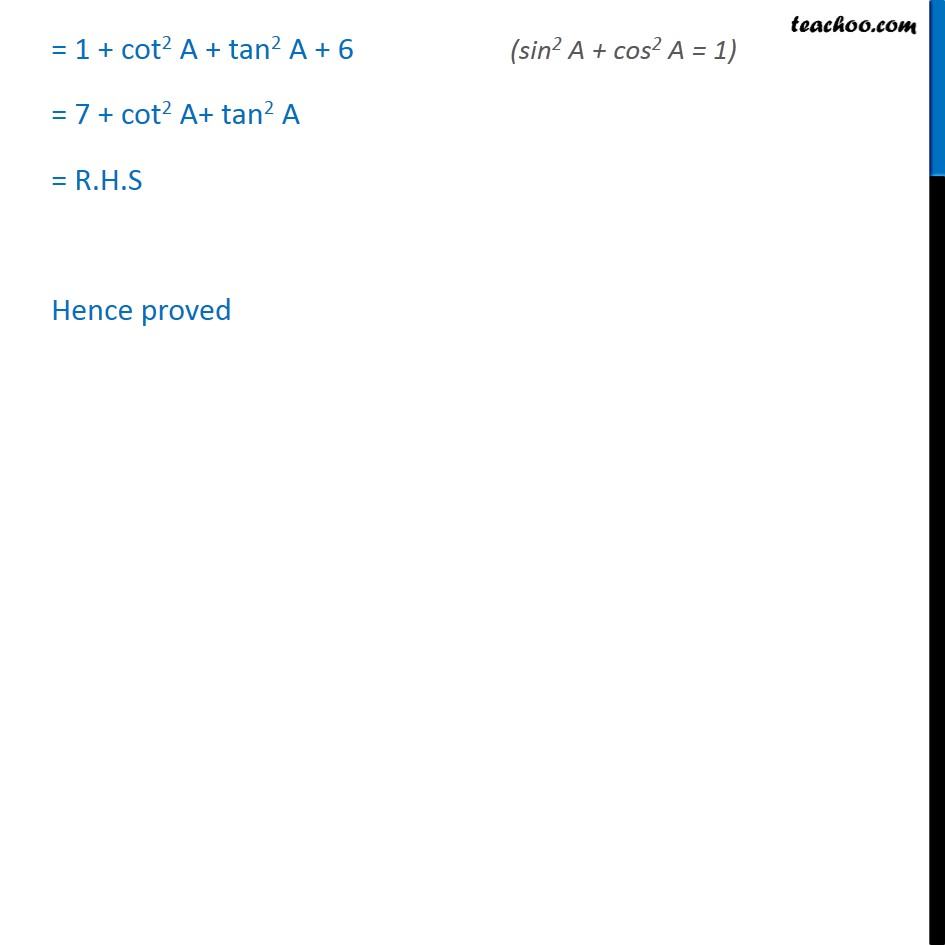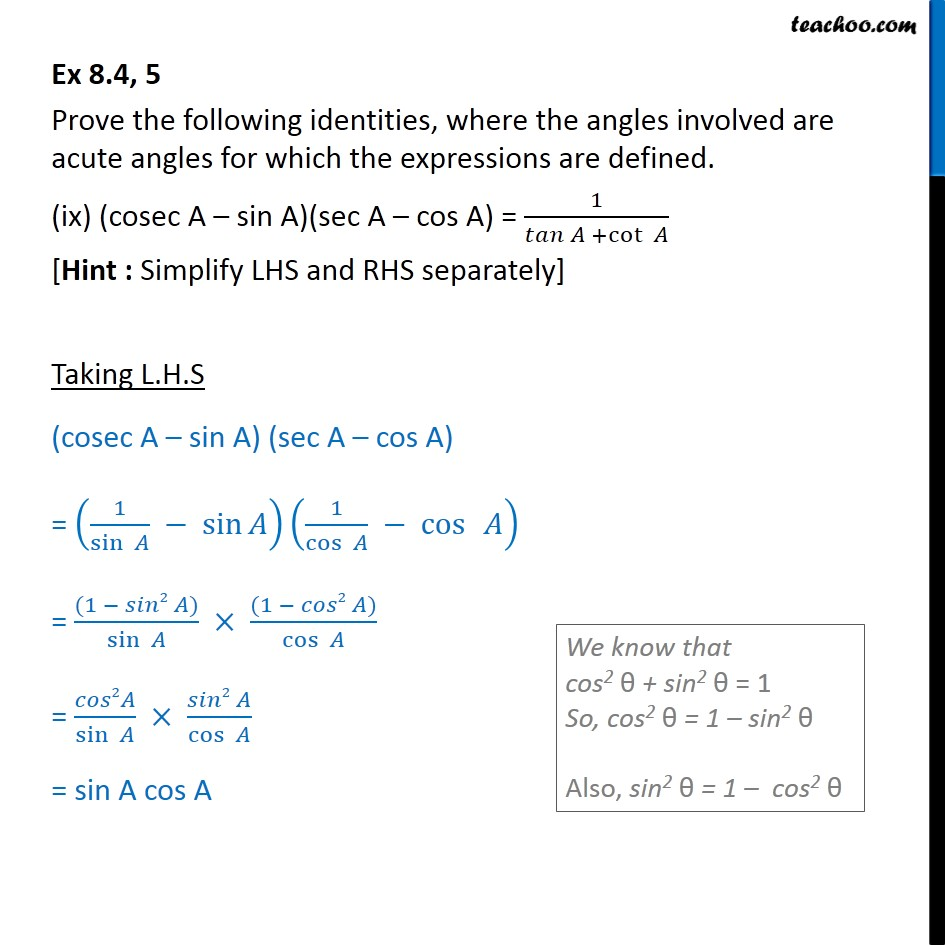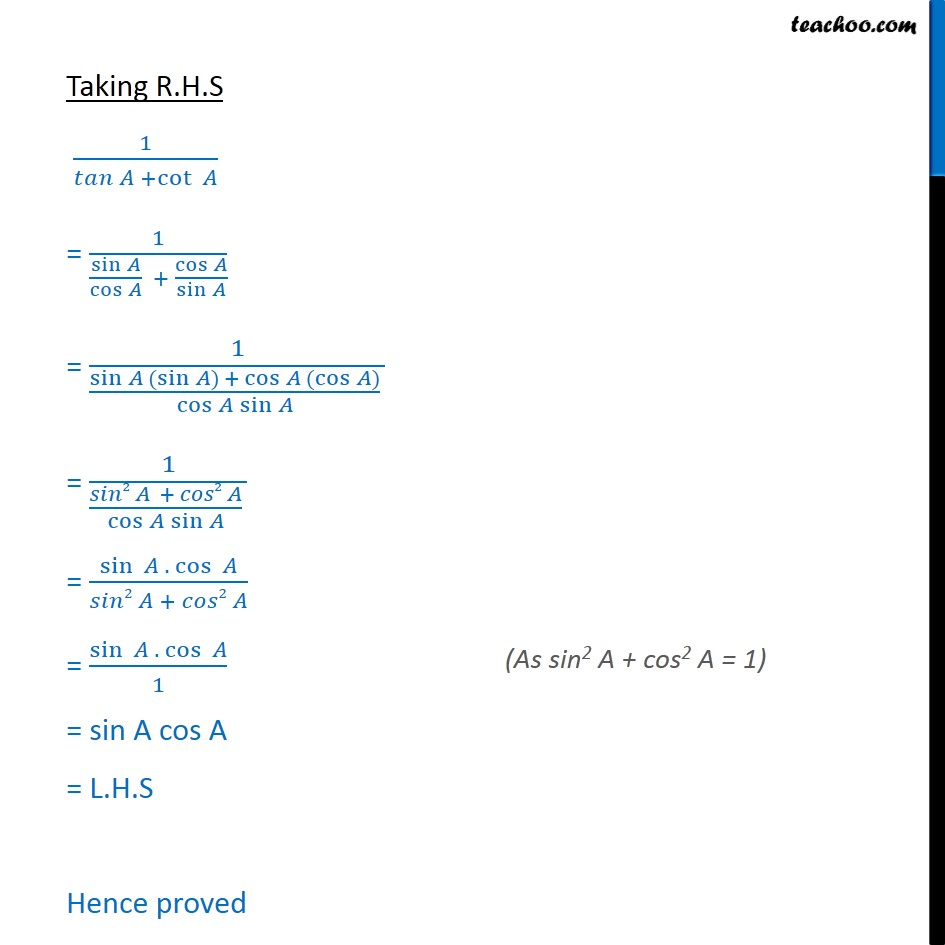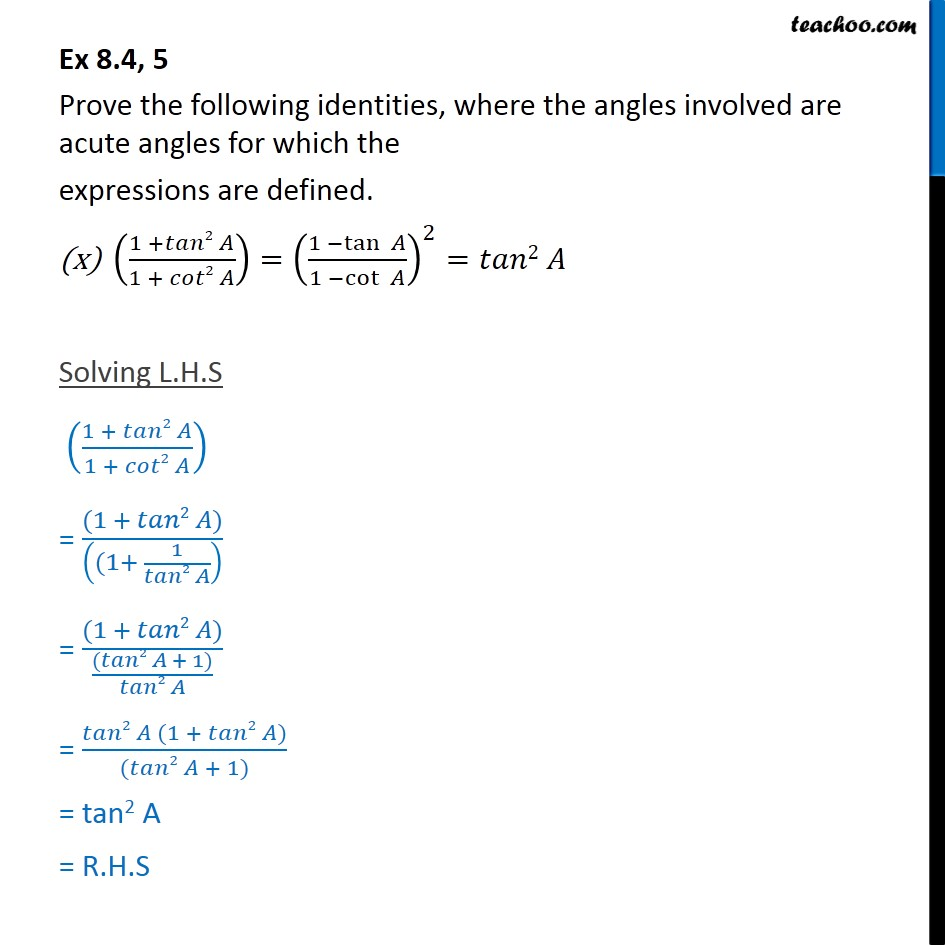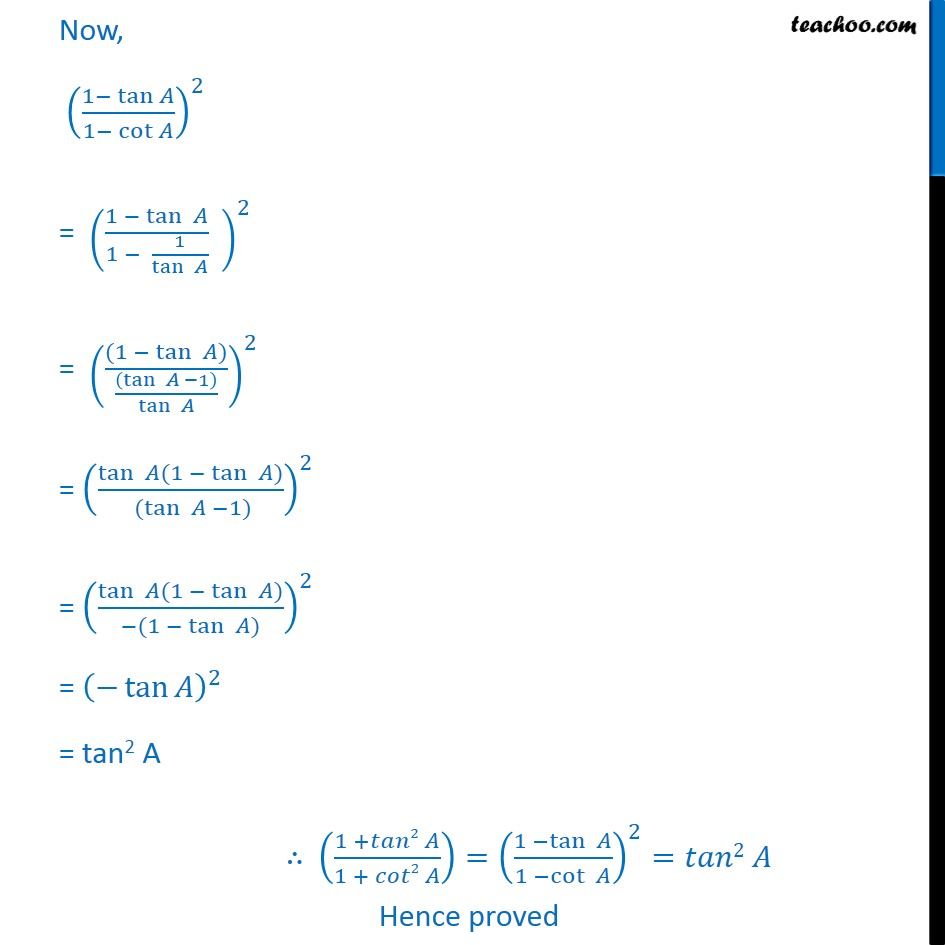1. Chapter 8 Class 10 Introduction to Trignometry
2. Serial order wise
3. Ex 8.4

Transcript

Ex 8.4, 5 Prove the following identities, where the angles involved are acute angles for which the expressions are defined. (i) (cosec cot )2 = (1 " " )/(1 + cos ) Solving L.H.S (cosec cot )2 We need to make it in terms of cos & sin = (1/sin cos /sin )^2 = (("1 " cos )/sin )^2 = ((1 cos )2 )/( 2 ) = ((1 cos )2 )/(1 2 ) = ((1 cos )2 )/(12 2 ) = (1 cos )^2/((1 + cos )(1 cos )) = ((1 cos )(1 ) )/((1 + cos )(1 cos )) = (1 cos )/(1 + cos ) = RHS Hence proved Ex 8.4, 5 Prove the following identities, where the angles involved are acute angles for which the expressions are defined. (ii) "cos A" /"1 + sin A" +"1 + sin A" /"cos A" =2 sec A Taking L.H.S (cos )/(1 + sin )+(1 + sin )/(cos ) = (cos (cos ) + (1 + sin )(1 + sin ) )/((1 + sin )(cos )) = ( 2 + (1 + sin )2)/((1 + sin )(cos )) = ( 2 + 1^2 + 2 + 2 sin )/((1 + sin )(cos )) = (( 2 + 2 ) + 1 + 2 sin )/((1 + sin )(cos ) ) = (1 + 1 + 2 sin )/((1 + sin )(cos ) ) = (2 + 2 sin )/((1 + sin )(cos ) ) = (2(1+ sin ))/((1 + sin )(cos ) ) = 2/(cos ) = 2 1/cos = 2 sec A = R.H.S L.H.S = R.H.S Hence proved Ex 8.4, 5 Prove the following identities, where the angles involved are acute angles for which the expressions are defined. (iii)tan /( 1 cot " " )+cot /(1 tan ) =1+ sec cosec [Hint : Write the expression in terms of sin and cos ] Taking L.H.S tan /( 1 cot " " )+cot /(1 tan ) = (sin /cos )/(1 (cos /sin ) )+((cos /sin ))/(1 (sin /cos ) ) = (sin /cos )/(((sin cos )/sin ) )+((cos /sin ))/( ((cos sin )/cos ) ) = sin /cos sin /(sin cos ) +cos /sin cos /(cos sin ) = sin2 /( cos ( sin cos ) ) + cos2 /( sin (cos sin ) ) = sin2 /( cos ( sin cos ) ) + cos2 /( sin ( sin cos ) ) = sin2 /( cos (sin cos ) ) cos2 /( sin ( sin cos ) ) = (sin2 (sin ) cos2 (cos ))/( cos ( sin cos )sin ) = (sin3 cos3 )/( cos sin ( sin cos ) ) = ( (sin cos ) (sin2 + cos2 +cos sin ))/( cos sin ( sin cos ) ) = ( (sin2 + cos2 +cos sin ))/(cos sin ) = ( (sin2 + cos2 ) + cos sin )/(cos sin ) = (1 + cos sin )/(cos sin ) = (1 )/(cos sin ) + (cos sin )/(cos sin ) = (1 )/cos (1 )/sin + 1 = sec cosec + 1 = 1 + sec cosec = R.H.S , L.H.S = R.H.S Hence proved Ex 8.4, 5 Prove the following identities, where the angles involved are acute angles for which the expressions are defined. ("1+sec" A)/"sec A" ="sin2 A" /"1 cos A" "[Hint : Simplify LHS and RHS separately]" Hence L.H.S = R.H.S Hence proved Ex 8.4, 5 Prove the following identities, where the angles involved are acute angles for which the expressions are defined. "cos A sin A + 1" /"cos A + sin A 1" = cosec A + cot A, using the identity cosec2 A = 1 + cot2 A. Taking L.H.S (cos sin + 1)/(cos + sin 1) divide both numerator and denominator by sin A = (1/sin (cos sin + 1 ))/(1/sin (cos + sin 1 ) ) = (cos /sin sin /sin + 1/sin )/(cos /sin + sin /sin 1/sin ) = cot 1 + /cot + 1 = ((cot + ) 1 )/((cot + 1 ) ) = ((co + ) ( 2 2 ) )/((cot + 1 )) = ((co + ) (cot )(cot + ) )/((cot + 1 )) = ((co + ) [1 ( )] )/([cot + 1 ]) = ((co + ) [1 + ] )/([cot + 1 ]) = cot A + cosec A = R.H.S Hence proved Ex 8.4, 5 Prove the following identities, where the angles involved are acute angles for which the expressions are defined. (vi) ((1 + sin )/(1 sin )) = sec A + tan A Taking L.H.S ((1 + sin )/(1 sin )) Rationalizing denominator Multiplying (1 + sin A) in numerator and denominator = (((1 + sin )(1 + sin ) )/((1 sin )(1 + sin ) )) = (((1 + sin )2 )/(12 2 )) = (((1 + sin )2 )/(1 2 )) = (((1 +sin )2 )/( 2 )) = (((1 + )/( ))^2 ) = (1 + sin )/cos = 1/cos + sin /cos = sec A + tan A = R.H.S Hence proved Ex 8.4, 5 Prove the following identities, where the angles involved are acute angles for which the expressions are defined. (vii) (sin 2 sin3 )/(2 cos3 cos )=tan Taking L.H.S (sin 2 sin3 )/(2 cos3 cos ) = (sin (1 2 sin2 ))/(cos (2cos2 1)) = sin /(cos ) ( (1 2sin2 ))/((2cos2 1)) = tan ( (1 2sin2 ))/((2cos2 1)) = tan ((1 2sin2 ))/((2(1 sin2 ) 1) ) = tan ((1 2sin2 ))/((2 2sin2 1)) = tan ((1 2sin2 ))/((1 2sin2 ) ) = tan 1 = tan = R.H.S Hence proved Ex 8.4, 5 Prove the following identities, where the angles involved are acute angles for which the expressions are defined. (sin A + cosec A)2 + (cos A + sec A)2 = 7 + tan2 A + cot2 A Taking L.H.S (sin A + cosec A)2 + (cos A + sec A)2 = (sin2 A + cosec2 A + 2sin A cosec A) + (cos2 A + sec2 A + 2 cos A . sec A) = (sin2 A + cosec2 A + 2sin A . 1/sin ) + (cos2 A + sec2 A + 2 cos A.1/cos ) = (sin2 A + cosec2 A + 2) + (cos2 A + sec2 A + 2) = (sin2 A + (1 + cot2 A) +2) + (cos2 A +(1 + tan2 A) + 2) = sin2 A + cos2 A + 1 + cot2 A + 2 + 1 + tan2 A + 2 = (sin2 A + cos2 A) + cot2 A + tan2 A + (1 + 2 + 1 + 2) = 1 + cot2 A + tan2 A + 6 = 7 + cot2 A+ tan2 A = R.H.S Hence proved Ex 8.4, 5 Prove the following identities, where the angles involved are acute angles for which the expressions are defined. (ix) (cosec A sin A)(sec A cos A) = 1/( +cot ) [Hint : Simplify LHS and RHS separately] Taking L.H.S (cosec A sin A) (sec A cos A) = (1/sin sin )(1/cos cos ) = ((1 2 ))/sin ((1 2 ))/cos = 2 /sin ( 2 )/cos = sin A cos A Taking R.H.S 1/( +cot ) = 1/(sin /cos + cos /sin ) = 1/(sin (sin ) + cos (cos ) /cos sin ) = 1/(( 2 + 2 )/cos sin ) = sin . cos /( 2 + 2 ) = sin . cos /1 = sin A cos A = L.H.S Hence proved Ex 8.4, 5 Prove the following identities, where the angles involved are acute angles for which the expressions are defined. ((1 + 2 )/(1 + 2 ))=((1 tan )/(1 cot ))^2= 2 Solving L.H.S ((1 + 2 )/(1 + 2 )) = ((1 + 2 ))/(((1+ 1/( 2 )) ) = ((1 + 2 ))/((( 2 + 1))/( 2 )) = ( 2 (1 + 2 ))/(( 2 + 1)) = tan2 A = R.H.S Now, ((1 tan )/(1 cot ))^2 = ((1 tan )/(1 1/tan ) " " )^2 = (((1 tan ) )/(((tan 1 ))/tan ))^2 = (tan (1 tan ) /( (tan 1) ))^2 = (tan (1 tan ) /( (1 tan ) ))^2 = ( tan )^2 = tan2 A

Ex 8.4